• +91 9971497814
• info@interviewmaterial.com

# RD Chapter 23- The Straight Lines Ex-23.8 Interview Questions Answers

### Related Subjects

Question 1 : A line passes through a point A (1, 2) and makes an angle of 600 with the x–axis and intercepts the line x + y = 6 at the point P. Find AP.

Given:

(x1, y1)= A (1, 2), θ = 60°

Let us find thedistance AP.

By using the formula,

The equation of theline is given by: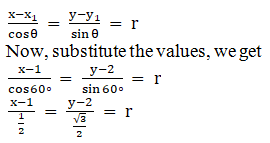Here, r represents thedistance of any point on the line from point A (1, 2).

The coordinate of anypoint P on this line are (1 + r/2, 2 + √3r/2)

It is clear that, Plies on the line x + y = 6

So,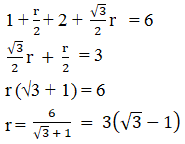The value of AP is3(√3 – 1)

Question 2 : If the straight line through the point P(3, 4) makes an angle π/6 with the x–axis and meets the line 12x + 5y + 10 = 0 at Q, find the length PQ.

Given:

(x1, y1)= A (3, 4), θ = π/6 = 30°

Let us find the lengthPQ.

By using the formula,

The equation of theline is given by: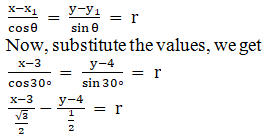x – √3 y + 4√3 – 3 = 0

Let PQ = r

Then, the coordinateof Q are given by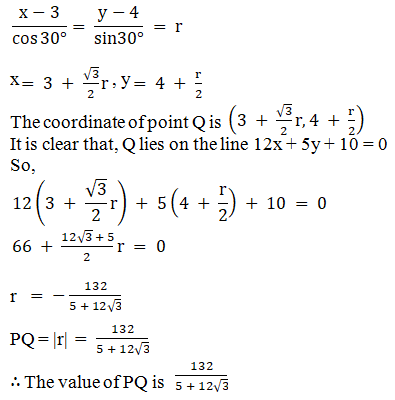Question 3 :

A straight line drawn through the point A (2, 1) making anangle π/4 with positive x–axis intersects another line x + 2y + 1 = 0in the point B. Find length AB.

Given:

(x1, y1)= A (2, 1), θ = π/4 = 45°

Let us find the lengthAB.

By using the formula,

The equation of theline is given by: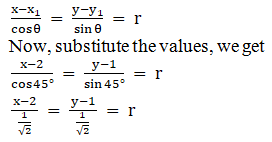x – y – 1 = 0

Let AB = r

Then, the coordinateof B is given by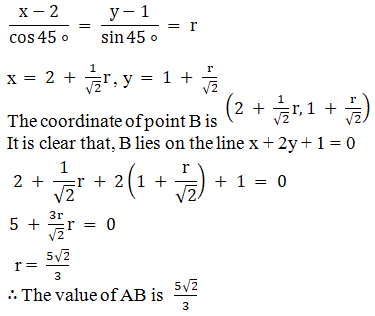Question 4 :

A line a drawn through A (4, – 1) parallel to the line 3x – 4y + 1 = 0.Find the coordinates of the two points on this line which are at a distance of5 units from A.

Given:

(x1, y1)= A (4, -1)

Let us findCoordinates of the two points on this line which are at a distance of 5 unitsfrom A.

Given: Line 3x – 4y +1 = 0

4y = 3x + 1

y = 3x/4 + 1/4

Slope tan θ = 3/4

So,

Sin θ = 3/5

Cos θ = 4/5

The equation of theline passing through A (4, −1) and having slope ¾ is

By using the formula,

The equation of theline is given by: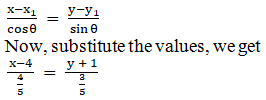3x – 4y = 16

Here, AP = r = ± 5

Thus, the coordinatesof P are given by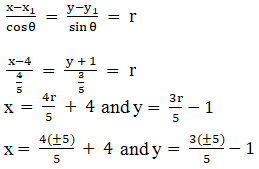x
= ±4 + 4 and y = ±3 –1

x = 8, 0 and y = 2, –4

The coordinates ofthe two points at a distance of 5 units from A are (8, 2) and (0, −4).

Question 5 : The straight line through P(x1, y1) inclined at an angle θ with the x–axis meets the line ax + by + c = 0 in Q. Find the length of PQ.

Given:

The equation of theline that passes through P(x1, y1) and makes anangle of θ with the x–axis.

Let us find the lengthof PQ.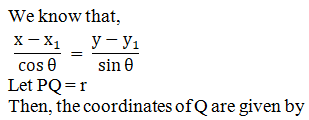By using the formula,

The equation of theline is given by: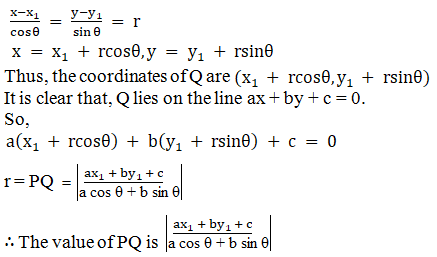Todays Deals### RD Chapter 23- The Straight Lines Ex-23.8 Contributorskrishan

Name:
Email:

# Latest News# 9000 interview questions in different categories